Atomic Structure

# 59 Electron Spin

### Learning Objectives

By the end of this section, you will be able to:

• Express the state of an electron in a hydrogen atom in terms of five quantum numbers
• Use quantum numbers to calculate the magnitude and direction of the spin and magnetic moment of an electron
• Explain the fine and hyperfine structure of the hydrogen spectrum in terms of magnetic interactions inside the hydrogen atom

In this section, we consider the effects of electron spin. Spin introduces two additional quantum numbers to our model of the hydrogen atom. Both were discovered by looking at the fine structure of atomic spectra. Spin is a fundamental characteristic of all particles, not just electrons, and is analogous to the intrinsic spin of extended bodies about their own axes, such as the daily rotation of Earth.

Spin is quantized in the same manner as orbital angular momentum. It has been found that the magnitude of the intrinsic spin angular momentum S of an electron is given by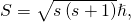where s is defined to be the spin quantum number. This is similar to the quantization of L given in (Figure), except that the only value allowed for s for an electron is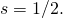The electron is said to be a “spin-half particle.” The spin projection quantum number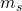is associated with the z-components of spin, expressed by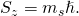In general, the allowed quantum numbers are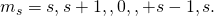For the special case of an electron (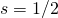),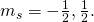Directions of intrinsic spin are quantized, just as they were for orbital angular momentum. The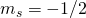state is called the “spin-down” state and has a z-component of spin,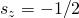;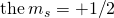state is called the “spin-up” state and has a z-component of spin,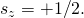These states are shown in (Figure).

The two possible states of electron spin.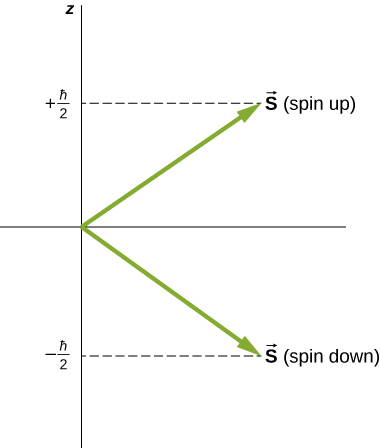The intrinsic magnetic dipole moment of an electron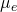can also be expressed in terms of the spin quantum number. In analogy to the orbital angular momentum, the magnitude of the electron magnetic moment is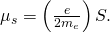According to the special theory of relativity, this value is low by a factor of 2. Thus, in vector form, the spin magnetic moment is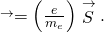The z-component of the magnetic moment is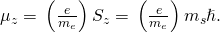The spin projection quantum number has just two values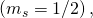so the z-component of the magnetic moment also has just two values: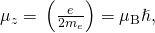where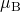is one Bohr magneton. An electron is magnetic, so we expect the electron to interact with other magnetic fields. We consider two special cases: the interaction of a free electron with an external (nonuniform) magnetic field, and an electron in a hydrogen atom with a magnetic field produced by the orbital angular momentum of the electron.

Electron Spin and Radiation A hydrogen atom in the ground state is placed in an external uniform magnetic field (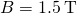). Determine the frequency of radiation produced in a transition between the spin-up and spin-down states of the electron.

Strategy The spin projection quantum number is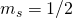, so the z-component of the magnetic moment is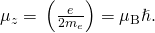The potential energy associated with the interaction between the electron magnetic moment and the external magnetic field is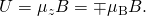The frequency of light emitted is proportional to the energy (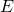) difference between these two states.

Solution The energy difference between these states is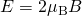, so the frequency of radiation produced is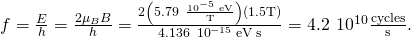Significance The electron magnetic moment couples with the external magnetic field. The energy of this system is different whether the electron is aligned or not with the proton. The frequency of radiation produced by a transition between these states is proportional to the energy difference. If we double the strength of the magnetic field, holding all other things constant, the frequency of the radiation doubles and its wavelength is cut in half.

In a hydrogen atom, the electron magnetic moment can interact with the magnetic field produced by the orbital angular momentum of the electron, a phenomenon called spin-orbit coupling. The orbital angular momentum (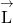), orbital magnetic moment (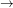), spin angular momentum (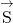), and spin magnetic moment (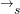) vectors are shown together in (Figure).

Just as the energy levels of a hydrogen atom can be split by an external magnetic field, so too are the energy levels of a hydrogen atom split by internal magnetic fields of the atom. If the magnetic moment of the electron and orbital magnetic moment of the electron are antiparallel, the potential energy from the magnetic interaction is relatively high, but when these moments are parallel, the potential energy is relatively small. Transition from each of these two states to a lower-energy level results in the emission of a photon of slightly different frequency. That is, the spin-orbit coupling “splits” the spectral line expected from a spin-less electron. The fine structure of the hydrogen spectrum is explained by spin-orbit coupling.

Spin-orbit coupling is the interaction of an electron’s spin magnetic momentwith its orbital magnetic moment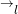.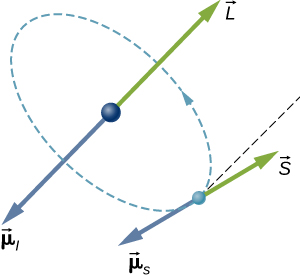The Stern-Gerlach experiment provides experimental evidence that electrons have spin angular momentum. The experiment passes a stream of silver (Ag) atoms through an external, nonuniform magnetic field. The Ag atom has an orbital angular momentum of zero and contains a single unpaired electron in the outer shell. Therefore, the total angular momentum of the Ag atom is due entirely to the spin of the outer electron (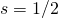. Due to electron spin, the Ag atoms act as tiny magnets as they pass through the magnetic field. These “magnets” have two possible orientations, which correspond to the spin-up and -down states of the electron. The magnetic field diverts the spin up atoms in one direction and the spin-down atoms in another direction. This produces two distinct bands on a screen ((Figure)).

In the Stern-Gerlach experiment, an external, nonuniform magnetic field diverts a beam of electrons in two different directions. This result is due to the quantization of spin angular momentum.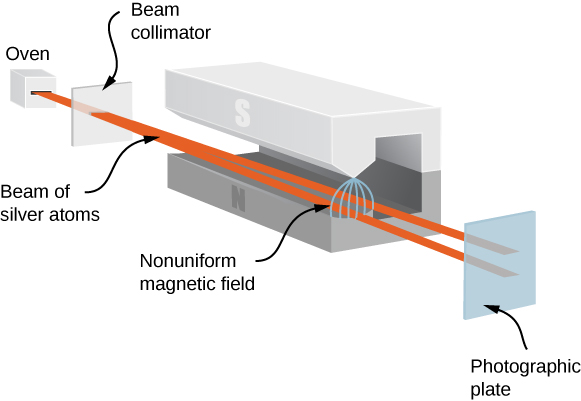According to classical predictions, the angular momentum (and, therefore, the magnetic moment) of the Ag atom can point in any direction, so one expects, instead, a continuous smudge on the screen. The resulting two bands of the Stern-Gerlach experiment provide startling support for the ideas of quantum mechanics.

Check Your Understanding If the Stern-Gerlach experiment yielded four distinct bands instead of two, what might be concluded about the spin quantum number of the charged particle?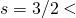Just like an electron, a proton is spin 1/2 and has a magnetic moment. (According to nuclear theory, this moment is due to the orbital motion of quarks within the proton.) The hyperfine structure of the hydrogen spectrum is explained by the interaction between the magnetic moment of the proton and the magnetic moment of the electron, an interaction known as spin-spin coupling. The energy of the electron-proton system is different depending on whether or not the moments are aligned. Transitions between these states (spin-flip transitions) result in the emission of a photon with a wavelength of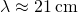(in the radio range). The 21-cm line in atomic spectroscopy is a “fingerprint” of hydrogen gas. Astronomers exploit this spectral line to map the spiral arms of galaxies, which are composed mostly of hydrogen ((Figure)).

The magnetic interaction between the electron and proton in the hydrogen atom is used to map the spiral arms of the Pinwheel Galaxy (NGC 5457). (a) The galaxy seen in visible light; (b) the galaxy seen in 21-cm hydrogen radiation; (c) the composite image of (a) and (b). Notice how the hydrogen emission penetrates dust in the galaxy to show the spiral arms very clearly, whereas the galactic nucleus shows up better in visible light (credit a: modification of work by ESA & NASA; credit b: modification of work by Fabian Walter).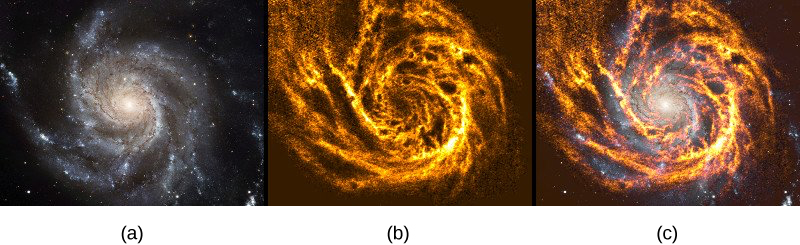A complete specification of the state of an electron in a hydrogen atom requires five quantum numbers: n, l, m, s, and. The names, symbols, and allowed values of these quantum numbers are summarized in (Figure).

Summary of Quantum Numbers of an Electron in a Hydrogen Atom
Name Symbol Allowed values
Principal quantum number n 1, 2, 3, …
Angular momentum l 0, 1, 2, … n – 1
Angular momentum projection m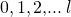Spin s 1/2 (electrons)
Spin projection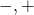Note that the intrinsic quantum numbers introduced in this section (s and ms) are valid for many particles, not just electrons. For example, quarks within an atomic nucleus are also spin-half particles. As we will see later, quantum numbers help to classify subatomic particles and enter into scientific models that attempt to explain how the universe works.

### Summary

• The state of an electron in a hydrogen atom can be expressed in terms of five quantum numbers.
• The spin angular momentum quantum of an electron is =. The spin angular momentum projection quantum number is ms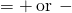(spin up or spin down).
• The fine and hyperfine structures of the hydrogen spectrum are explained by magnetic interactions within the atom.

### Conceptual Questions

Explain how a hydrogen atom in the ground state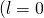) can interact magnetically with an external magnetic field.

Even in the ground state (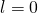), a hydrogen atom has magnetic properties due the intrinsic (internal) electron spin. The magnetic moment of an electron is proportional to its spin.

Compare orbital angular momentum with spin angular momentum of an electron in the hydrogen atom.

List all the possible values of s andfor an electron. Are there particles for which these values are different?

For all electrons,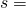and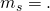As we will see, not all particles have the same spin quantum number. For example, a photon as a spin 1 (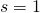), and a Higgs boson has spin 0 (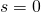).

Are the angular momentum vectors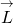and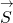necessarily aligned?

What is spin-orbit coupling?

An electron has a magnetic moment associated with its intrinsic (internal) spin. Spin-orbit coupling occurs when this interacts with the magnetic field produced by the orbital angular momentum of the electron.

### Problems

What is the magnitude of the spin momentum of an electron? (Express you answer in terms of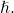What are the possible polar orientations of the spin momentum vector for an electron?

Spin up (relative to positive z-axis):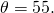Spin down (relative to positive z-axis):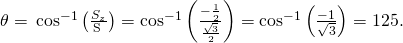For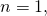write all the possible sets of quantum numbers (n, l, m,).

A hydrogen atom is placed in an external uniform magnetic field (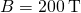). Calculate the wavelength of light produced in a transition from a spin up to spin down state.

The spin projection quantum number is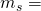, so the z-component of the magnetic moment is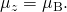The potential energy associated with the interaction between the electron and the external magnetic field is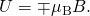The energy difference between these states is, so the wavelength of light produced is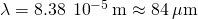If the magnetic field in the preceding problem is quadrupled, what happens to the wavelength of light produced in a transition from a spin up to spin down state?

If the magnetic moment in the preceding problem is doubled, what happens to the frequency of light produced in a transition from a spin-up to spin-down state?

It is increased by a factor of 2.

For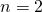, write all the possible sets of quantum numbers (n, l, m,).

### Glossary

fine structure
detailed structure of atomic spectra produced by spin-orbit coupling
hyperfine structure
detailed structure of atomic spectra produced by spin-orbit coupling
spin-flip transitions
atomic transitions between states of an electron-proton system in which the magnetic moments are aligned and not aligned
spin-orbit coupling
interaction between the electron magnetic moment and the magnetic field produced by the orbital angular momentum of the electron
spin projection quantum number ()
quantum number associated with the z-component of the spin angular momentum of an electron
spin quantum number (s)
quantum number associated with the spin angular momentum of an electron